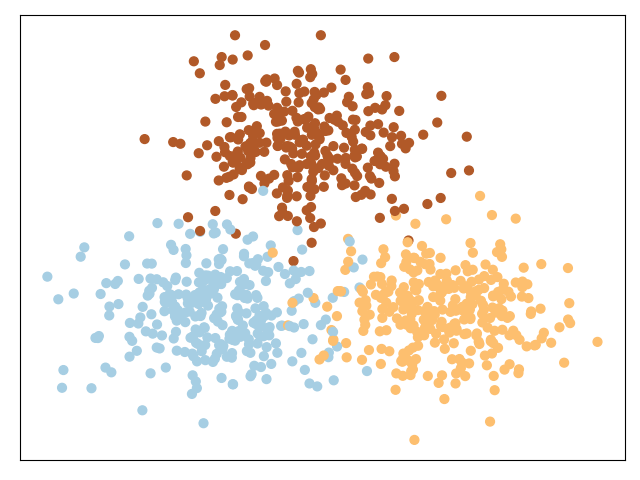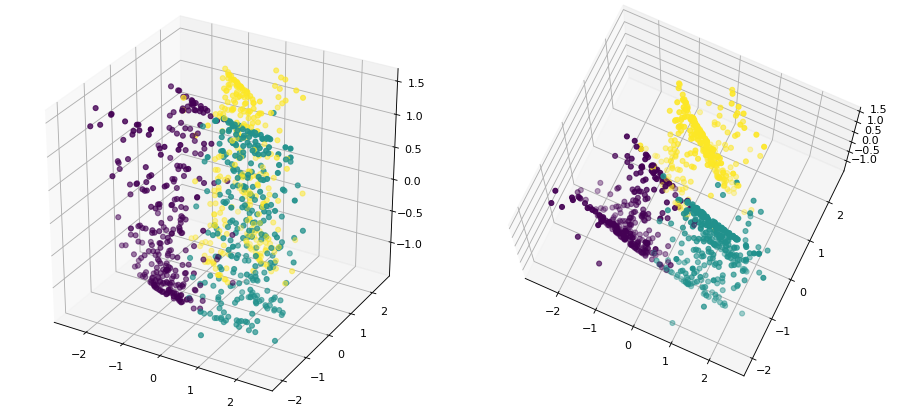# WKmeans一种基于特征权重的聚类算法

### 1 引例## 2 加权KMeans聚类算法

d x c 1 = 0.49 × ( 4 − 2 ) 2 + 0.49 × ( 4 − 3 ) 2 + 0.02 × ( 1 − 2 ) 2 = 2.47 d x c 2 = 0.49 × ( 4 − 3 ) 2 + 0.49 × ( 4 − 5 ) 2 + 0.02 × ( 1 − 9 ) 2 = 2.26 (1) \begin{aligned} d_{xc_1}=0.49\times(4-2)^2+0.49\times(4-3)^2+0.02\times(1-2)^2=2.47\\[2ex] d_{xc_2}=0.49\times(4-3)^2+0.49\times(4-5)^2+0.02\times(1-9)^2=2.26 \end{aligned}\tag{1}

### 2.1 WKmeans原理

P ( U , Z , W ) = ∑ p = 1 k ∑ i = 1 n u i p ∑ j = 1 m w j β ( x i j − z p j ) 2 (2) P(U,Z,W)=\sum_{p=1}^k\sum_{i=1}^nu_{ip}\sum_{j=1}^mw^{\beta}_j(x_{ij}-z{pj})^2\tag{2}

∑ j = 1 m w j = 1 (3) \sum_{j=1}^mw_j=1\tag{3}

### 2.2 WKmeans迭代公式

• 簇分配矩阵 U U
u i p = { 1 , w j β ∑ j = 1 m ( x i j − z p j ) 2 ≤ w j β ∑ j = 1 m ( x i j − z t j ) 2 ,    for  1 ≤ t ≤ k 0 , otherwise (4) u_{ip}= \begin{cases} 1, & w^{\beta}_j\sum\limits_{j=1}^m(x_{ij}-z_{pj})^2\leq w^{\beta}_j\sum\limits_{j=1}^m(x_{ij}-z_{tj})^2,\;\text{for }1\leq t \leq k\\[1ex] 0, & \text{otherwise} \end{cases}\tag{4}

• 簇中心矩阵 Z Z
z p j = ∑ i = 1 n u i p x i j ∑ i = 1 n u i p (5) z_{pj}=\frac{\sum\limits_{i=1}^nu_{ip}x_{ij}}{\sum\limits_{i=1}^nu_{ip}} \tag{5}

• 权重矩阵 W W
w j = 1 ∑ t = 1 m [ D j D t ] 1 β − 1 ,      β > 1  or  β ≤ 0 (6) w_j=\frac{1}{\sum\limits_{t=1}^m\left[\frac{D_j}{D_t}\right]^{\frac{1}{\beta-1}}},\;\;\beta>1 \text{ or }\beta\leq0\tag{6}
其中
D j = ∑ p = 1 k ∑ i = 1 n u i p ( x i j − z p j ) 2 (7) D_j=\sum_{p=1}^k\sum_{i=1}^nu_{ip}(x_{ij}-z_{pj})^2\tag{7}
可以看出， D j D_j 其实就是所有样本点在第 j j 个维度上的距离和。

### 2.3 动手实现

def computeWeight(X, centroid, idx, K, belta):
n, m = X.shape
weight = np.zeros((1, m), dtype=float)
D = np.zeros((1, m), dtype=float)
for k in range(K):
index = np.where(idx == k)
temp = X[index, :]  # 取第k个簇的所有样本
distance2 = np.power((temp - centroid[k, :]), 2)  # ? by m
D = D + np.sum(distance2, axis=0)
e = 1 / float(belta - 1)
for j in range(m):
temp = D[j] / D
weight[j] = 1 / np.sum((np.power(temp, e)), axis=0)
return weight


if __name__ == '__main__':
x, y, x_noise = make_data()
y_pred = wkmeans(x, 3, belta=3)
nmi = normalized_mutual_info_score(y, y_pred)
print("NMI without noise: ", nmi)

y_pred = wkmeans(x_noise, 3,belta=3)
nmi = normalized_mutual_info_score(y, y_pred)
print("NMI with noise by ours: ", nmi)
#结果
NMI without noise:  0.867939056164429
NMI with noise:  0.852210021893048


### 2.4 参数求解

Φ ( W , α ) = ∑ j = 1 m w j β D j + α ( ∑ j = 1 m w j − 1 ) (8) \Phi(W,\alpha)=\sum_{j=1}^mw^{\beta}_jD_j+\alpha\left(\sum_{j=1}^mw_j-1\right) \tag{8}

∂ Φ ∂ w j = β w j β − 1 D j + α = 0 (9) \frac{\partial \Phi}{\partial w_j}=\beta w^{\beta-1}_jD_j+\alpha=0\\ \tag{9}

∂ Φ ∂ α = ∑ j = 1 m w j − 1 = 0 (10) \frac{\partial \Phi}{\partial \alpha}=\sum_{j=1}^mw_j-1=0\tag{10}

w j = ( − α β D j ) 1 β − 1 (11) w_j=\left(\frac{-\alpha}{\beta D_j}\right)^{\frac{1}{\beta-1}}\tag{11}
( 11 ) (11) 代入 ( 10 ) (10) 得：
∑ j = 1 m ( − α β D j ) 1 β − 1 = 1 (12) \sum_{j=1}^m\left(\frac{-\alpha}{\beta D_j}\right)^{\frac{1}{\beta-1}}=1\tag{12}

( − α ) 1 β − 1 = 1 / [ ∑ t = 1 m ( 1 β D t ) 1 β − 1 ] (13) (-\alpha)^{\frac{1}{\beta-1}}=1/\left[\sum_{t=1}^m\left(\frac{1}{\beta D_t}\right)^{\frac{1}{\beta-1}}\right]\tag{13}
( 13 ) (13) 代入 ( 11 ) (11) 即可得到：
w j = 1 ( β D j ) 1 β − 1 ∑ t = 1 m ( 1 β D t ) 1 β − 1 = 1 ( D j ) 1 β − 1 ∑ t = 1 m ( 1 D t ) 1 β − 1 = 1 ∑ t = 1 m ( D j D t ) 1 β − 1 (14) \begin{aligned} w_j=&\frac{1}{(\beta D_j)^{\frac{1}{\beta-1}}\sum\limits_{t=1}^m\left(\frac{1}{\beta D_t}\right)^{\frac{1}{\beta-1}}}\\[2ex] =&\frac{1}{(D_j)^{\frac{1}{\beta-1}}\sum\limits_{t=1}^m\left(\frac{1}{D_t}\right)^{\frac{1}{\beta-1}}}\\[2ex] =&\frac{1}{\sum\limits_{t=1}^m\left(\frac{D_j}{D_t}\right)^{\frac{1}{\beta-1}}} \end{aligned}\tag{14}

## 3 总结

### 引用

Automated Variable Weighting in k-Means Type Clustering 公众号回复“论文”即可获得下载链接

示例代码 ： https://github.com/moon-hotel/MachineLearningWithMe

WKmeans: https://github.com/moon-hotel/WKmeans

### 推荐阅读

Kmeans聚类算法

Kmeans++聚类算法

聚类评估指标07-122万+06-061094
05-168万+
07-022万+
06-23362
03-113万+
10-141343
06-157076
03-313883
12-1817万+
12-19
04-01
06-13
08-301万+
06-082288
05-185万+
08-10598
03-035065
12-042030
01-07112